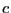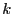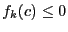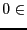Next: Example Up: Laguerre method Previous: Mathematical background   Contents

### Implementation

This procedure is able to give upper and lower bound on the value of the roots. In the implementation we defineas the smallest real which satisfy. If we findsuch that, then we increaseby a given positive value sens and start again. We limit the number of iteration of the scheme by giving a maximal value for the number of iteration:

int Laguerre_Bound_Interval(int Degree,VECTOR &Coeff,double sens,int MaxIter,double *bound);

with:
• Degree: degree of the polynomial
• Coeff: the Degree+1 coefficients of the polynomial in increasing degree
• sens: a positive real indicating the increase ofin the scheme
• MaxIter: the maximum number of iteration. If this number is exceeded the procedure returns 0.
• bound: the upper value of the real root
This procedure fail and returns 0 if Degree=0, Coeff(1)=0, if Coeff(Degree+1)=0 and if the number of iteration exceed MaxIter. On success the return code is 1. Note that the bound given by Laguerre (assuming that Coeff(Degree+1) is positive) cannot be lower than -Coeff(Degree)/Coeff(Degree+1).

The lower bound of the root may be determined by:


int Laguerre_Bound_Inverse_Interval(int Degree,VECTOR &Coeff1,double amp_sens,
int MaxIter,double *bound);

We have also a procedure which determine upper and lower bound for the real roots:

int Laguerre_Bound_Interval(int Degree,VECTOR &Coeff,double sens,int MaxIter,INTERVAL &Bound);

All the real roots lie within Bound. We may also use this procedure for interval polynomial:

int Laguerre_Bound_Interval(int Degree,INTERVAL_VECTOR &Coeff,double sens,
int MaxIter,INTERVAL &Bound);

This procedure fail and returns 0 if Degree=0,Coeff(1), ifCoeff(Degree+1) and if the number of iteration exceed MaxIter. If Bound=[a,b], then the real roots of all the polynomial in the set are lower than b and for some polynomial in the set the roots may be lower than a. A similar procedure exists for upper and lower bound.

int Laguerre_Bound_Interval(int Degree,INTERVAL_VECTOR &Coeff,double sens,
int MaxIter,INTERVAL &Lower,INTERVAL &Upper);

In that case Upper=[a,b] will be such that value of all roots of any polynomial within the set is lower than b, while for some polynomial they will be lower than a. On the other hand Lower=[a,b] will be such that the value of all roots of any polynomial within the set is greater than a, while for some polynomial they will be greater than b.Next: Example Up: Laguerre method Previous: Mathematical background   Contents
Jean-Pierre Merlet 2012-12-20